Journal DetailsFormat
Journal
eISSN
2083-134X
First Published
16 Apr 2011
Publication timeframe
4 times per year
Languages
EnglishOpen Access

# High accuracy computational methods for behavioral modeling of thick-film resistors at cryogenic temperatures

###### Accepted: 21 Jan 2016
Journal DetailsFormat
Journal
eISSN
2083-134X
First Published
16 Apr 2011
Publication timeframe
4 times per year
Languages
English

The aim of this work was to elaborate two-dimensional behavioral modeling method of thick-film resistors working in low-temperature conditions. The investigated resistors (made from 5 various resistive inks: 10 resistor coupons, each with 36 resistors with various dimensions), were measured automatically in a cryostat system. The low temperature was achieved in a nitrogen-helium continuous-flow cryostat. For nitrogen used as a freezing liquid the minimal temperature possible to achieve was equal to −195.85 °C (77.3 K). Mathematical model in the form of a multiplication of two polynomials was elaborated based on the above mentioned measurements. The first polynomial approximated temperature behavior of the normalized resistance, while the second one described the dependence of resistance on planar resistors dimensions. Special computational procedures for multidimensional approximation purpose were elaborated. It was shown that proper approximation polynomials and sufficiently exact methods of calculations ensure acceptable modeling errors.

#### Keywords

Introduction

Thick-film resistors have been used in hybrid microcircuits for about 50 years. However, there is still no full explanation of different physicochemical processes which occur during their fabrication and their relationships with resistor electrical properties because commercially available thick-film components are very complicated nonequilibrium systems. Every thick-film resistive ink consists of four subsystems – metallic (conductive) phase, glass, organic vehicle and modifiers, which should be deposited on a proper substrate. During firing there are physicochemical, thermodynamical and mechanical interactions inside the mentioned subsystems or among them, the substrates and terminations. The knowledge about such interactions makes possible to obtain passive elements with assumed exploitation parameters [1, 2]. For example, a change of firing profile, topology and/or terminations metallurgy leads to intentional change of resistance-temperature characteristics of a specified resistor (Fig. 1), e.g. by shifting the minimum of R(T) characteristics to the desired temperature range of operation of an electrical circuit. But a very simple qualitative DC electrical equivalent circuit of a surface or buried thick-film resistor (Fig. 1) suggests that both processes in the resistor volume (Rb) as well as in the interface region between the resistive film and terminations (Rk) affect R(T) characteristics. It is thus clear that the interface processes become more and more important for modern miniaturized components.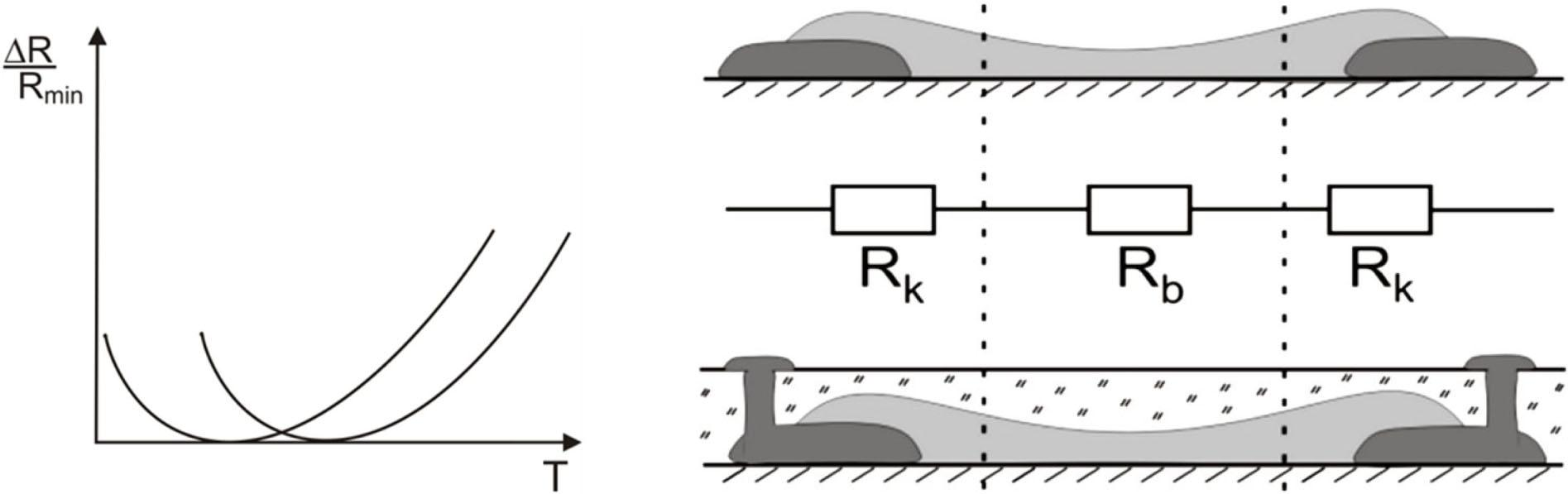Typical shape of R(T) characteristics of thick-film resistor (left) and a simple DC electrical equivalent circuit for the surface and buried resistor (right).

On the other hand, the temperature properties of thick-film resistors are important for designing and operation of electronic circuits for military, medical or outer space applications as well as for wide temperature range circuits . For example, some recommendations for the application of film resistors in low-temperature electronic circuits can be found in . In general, the temperature properties of thick-film resistors are well described in a standard temperature range (between –55 °C and 125 °C). Also their R(T) characteristics, from very low up to room temperature (–190 °C ≤ T ≤ 25 °C or even higher) are very important for the analysis of possible conduction mechanisms in such composites [5-13]. However, such models are not recommended for designers and users of electronic circuits because of their complexity .

The mathematical models, worked out on the basis of measurements, are a good solution to this situation. Therefore, the aim of this paper was to elaborate two-dimensional mathematical (behavioral) modeling method for thick-film resistors working in low-temperature and at DC or low frequency conditions (with neglected parasitic elements) for circuit design purposes. One should notice that this attempt is different from that applied by manufacturers of cryogenic temperature sensors and controllers. For example Lake Shore Cryotronics, Inc., offers temperature sensors in three versions: not-calibrated, abbreviate callibrated, e.g. at two or three points, and fully calibrated . The calibrated sensors are provided among others with a table containing calibration data (resistance vs. temperature) and a curve fit allowing calculation of temperature from the measurement of resistance T(R). Two methods of approximation are there used: the first curve fit type is a polynomial equation based on Chebyshev polynomials; the second one is based on cubic spline route. Both methods are done in chosen narrow temperature subranges and recursively generated Chebyshev polynomials are determined for normalized resistances (resistances divided by the resistance subranges).

Authors of paper  proposed to describe the T(R) dependence using the following formula:

$1T=A+Bln(R−R0)+Cln2(R−R0)+Dln3(R−R0)$$$\matrix{ {{1 \over {\sqrt T }} = A + B\ln \left( {R - {R_0}} \right) + C{{\ln }^2}\left( {R - {R_0}} \right)} \cr { + D{{\ln }^3}\left( {R - {R_0}} \right)} \cr }$$

which provides a very good description of temperature T of the thermometer analyzed by author as a function of device resistance R. R0 is the offset resistance whose value can be chosen so that the residual errors were not larger than 10 K in the whole temperature range even up to room temperature.

For our purposes, the mathematical model needs the temperature and dimensional dependence of resistance R(T,l,w) to be described in a symbolic form. The curve fit methods described above concern inverse temperature characteristics T(R). But in our modeling, R(T) in an explicit (developed) form is needed. The methods mentioned above cannot be applied for derivation of the R(T) relationship because of lack of the unique solutions. The purpose of our work was to develop the mathematical models of thick-film resistors to improve the accuracy of electronic circuit design, in the range of –200 °C to +25 °C. In this temperature range direct polynomial approximation appeared to be sufficiently exact.

The resistor models with a high accuracy are needed for designing high accuracy electronic circuits such as e.g. high accuracy amplifiers (instrumentation amplifiers), small measuring bridges, thermal compensation systems, notch filters and so on. In a notch filter , consisting of a bandpass filter and a summer, the gain at the center frequency of the bandpass filter through the summer must be equal to the input gain through the summer. The more closely the two gains match, the deeper the notch. Therefore, appropriate resistors should be designed very precisely (Exact calculations show that for scattering of a filter gain smaller than 10 % the resistors must demonstrate smaller than 5 % scattering of resistance). Therefore, we paid special attention to the model accuracy and its temperature dependence. The mathematical models in the form of a multiplication of two 1D-and 2D-polynomials were elaborated for 5 types of thick-film resistors. The paper is organized as follows: in Section 2, the measurement and data collecting system is described, while in Section 3 the modeling method is explained; Section 4 contains description of the modeling results.

Experimental
Measurement system

At first, instrument set-up and measurement methods needed to collect the necessary data have been worked out. A cryostat system for characterization of electronic components and circuits in low-temperature conditions was used . This system exploits the continuous gas-flow type N2/He cryostat working under LabVIEW program control. The principle of its operation is shown in Fig. 2.

The liquid nitrogen (LN2)or helium (LHe) can be used as a cooling liquid. The source of LN2 is a Dewar vessel from which the liquid is transported to the cryostat chamber through a siphon with a siphon bulb. Liquid fumes are transported from the chamber to the heater, tested devices (DUT), flow meter and finally to the outlet of fumes. There are also two minor installations used for pressure control inside the Dewar vessel and for achieving vacuum in the cryostat chamber sheath. The resistance temperature sensor and PID regulator were applied for temperature control. The test resistors were made from three Polish (R323, R324, R325) and two Du Pont (DP1931, DP1939) inks with 1, 10 or 100 kΩ/sq sheet resistances, respectively. Ten test coupons consisted of 36 thick-film resistors printed on 30 × 50 mm2 alumina substrate (96 % Al2O3)and terminations with PdAg conductors were made from each paste. The conductors and resistors were screen-printed with a 200 mesh screen and fired under a standard temperature profile with 850 °C peak temperature in a 60 min cycle. All pair combinations from the following set of values: (0.59, 1.31, 2.54, 3.96, 5.19, 5.91) (mm) were used as the length and width of the designed resistors. To measure R(T) characteristics the resistor arrays were placed in a special probe holder with gold pins (Fig. 3) mounted inside the cryostat and connected with measuring instruments using appropriate cables and connectors.

The measurements were performed in a wide temperature range between 180 °C and +20 °C. All measurements were performed under NI Lab-VIEW software and GPIB interface with the highest possible accuracy. To avoid parasitic effects, short-and open-circuit corrections were made at the beginning of measurements. The resistance was measured at 100 kHz and 1 V amplitude using HP4263A LCR meter with $512$$5{1 \over 2}$ digits accuracy. For minimizing uncertainty in measurements, the result of measurement was calculated as an average value of 64 measurements taken at one point. The resistors were switched by a Keithley 7001 scanner.

Data collecting

The measured resistances R were presented in a matrix form at each temperature (Table 1). Every element of this matrix (resistance) is a certain mathematical function of temperature. The typical plots of mean-value resistance and standard deviation are shown in Fig. 4. In further considerations we took these characteristics averaged over 10 substrates and all 36 resistors (so-called mean-value characteristics). The points in Fig. 4 and Fig. 5 correspond to measurement points, whereas continuous lines have been obtained from the fitting procedure. Red and green lines in Fig. 4 represent resistance characteristics approximated by polynomial plus standard deviation (R +s) and polynomial minus standard deviation (R – s). In this way, the possible area of characteristics dispersion has been depicted. The semirelative temperature sensitivity characteristics: the mean value of a differential temperature coefficient of resistance (TCR) is defined as:

$sem_TCR=(1/R)dR(T)/dT$$$sem\_TCR = \left( {1/R} \right)dR\left( T \right)/dT$$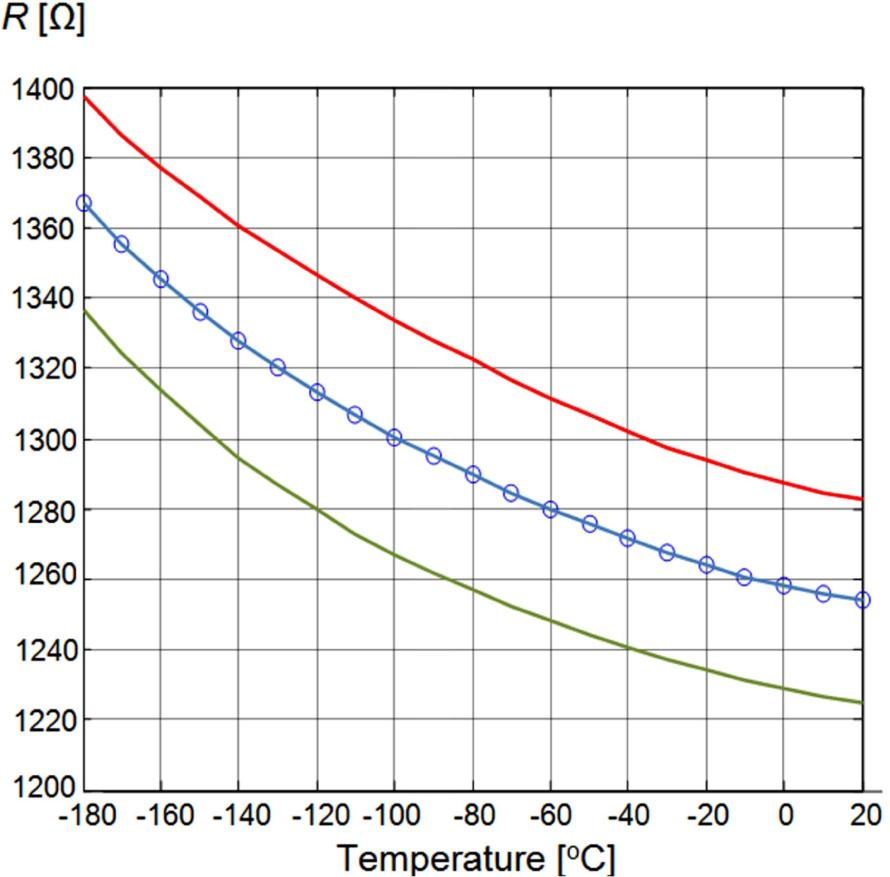Resistance and its standard deviation vs. temperature (R323): a dotted blue line – mean-value resistance measured R, b red and green lines – +/-standard deviation of resistance.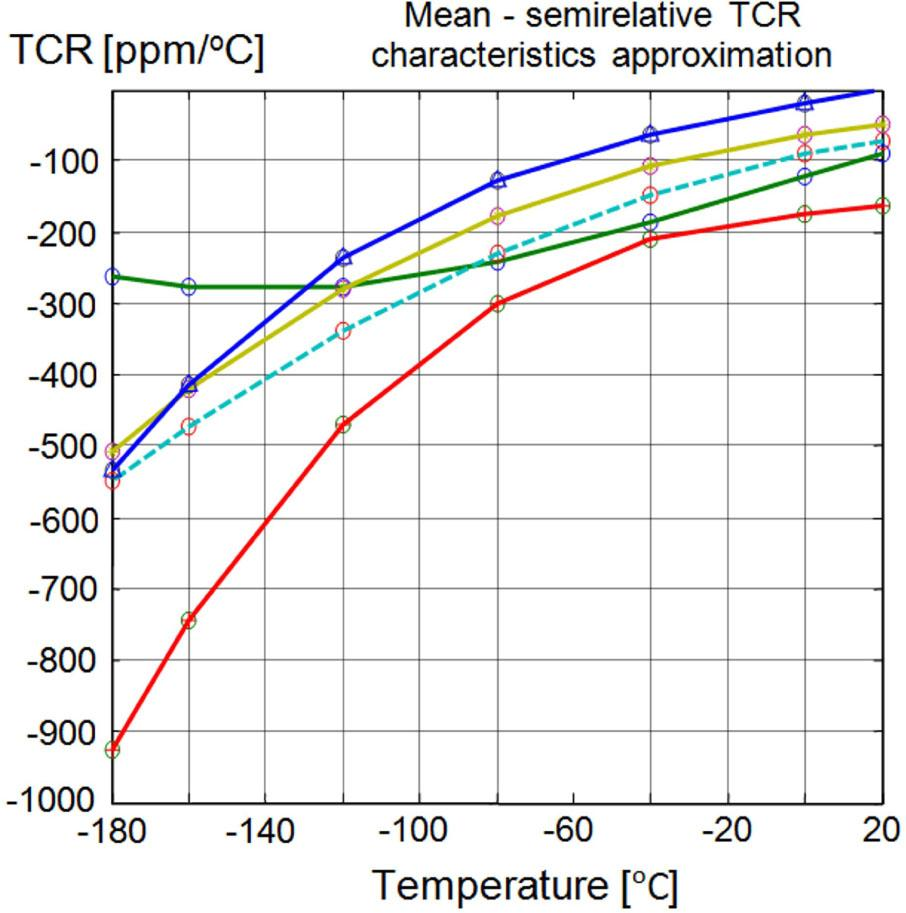Mean-value differential TCR characteristics vs. temperature, R323 – green line, R324 – light blue dotted line, R325 – yellow line, DP1931 – blue line, DP1939 – red line.

Matrix of resistances for every test coupon at each temperature.

[mm]w = 5.91w = 5.19w = 3.96w = 2.51w = 1.31w = 0.59
l = 5.91R1(T)R2(T)R3(T)R4(T)R5(T)R6(T)
l = 5.19R7(T)R8(T)R9(T)R10(T)R11(T)R12(T)
l = 3.96R13(T)R14(T)R15(T)R16(T)R17(T)R18(T)
l = 2.51R19(T)R20(T)R21(T)R22(T)R23(T)R24(T)
l = 1.31R25(T)R26(T)R27(T)R28(T)R29(T)R30(T)
l = 0.59R31(T)R32(T)R33(T)R34(T)R35(T)R36(T)

Based on this definition, the measured TCR results are shown in Fig. 5. As we can see, the TCR is temperature dependent in the considered wide temperature range. All tested resistors have a negative TCR in the studied temperature range. Moreover, the resistors from higher-resistive inks (DP1939 – red line) exhibit larger resistance increase when the temperature is decreased (Fig. 6) and more negative TCR (Fig. 5) than the components from lower-resistive inks (DP1931 – blue line). This observation is in agreement with the data presented in  for SMD 0805 thick-film resistors from YA-GEO. The temperature dependence of normalized Rr(T) =R(T)/R0 (Fig. 6), (for instance R0 – resistance at 0 °C) seems to be more universal than the typical temperature characteristics. Based on the results presented above we decided to fit the normalized resistance dependence as n–th order polynomial function of temperature.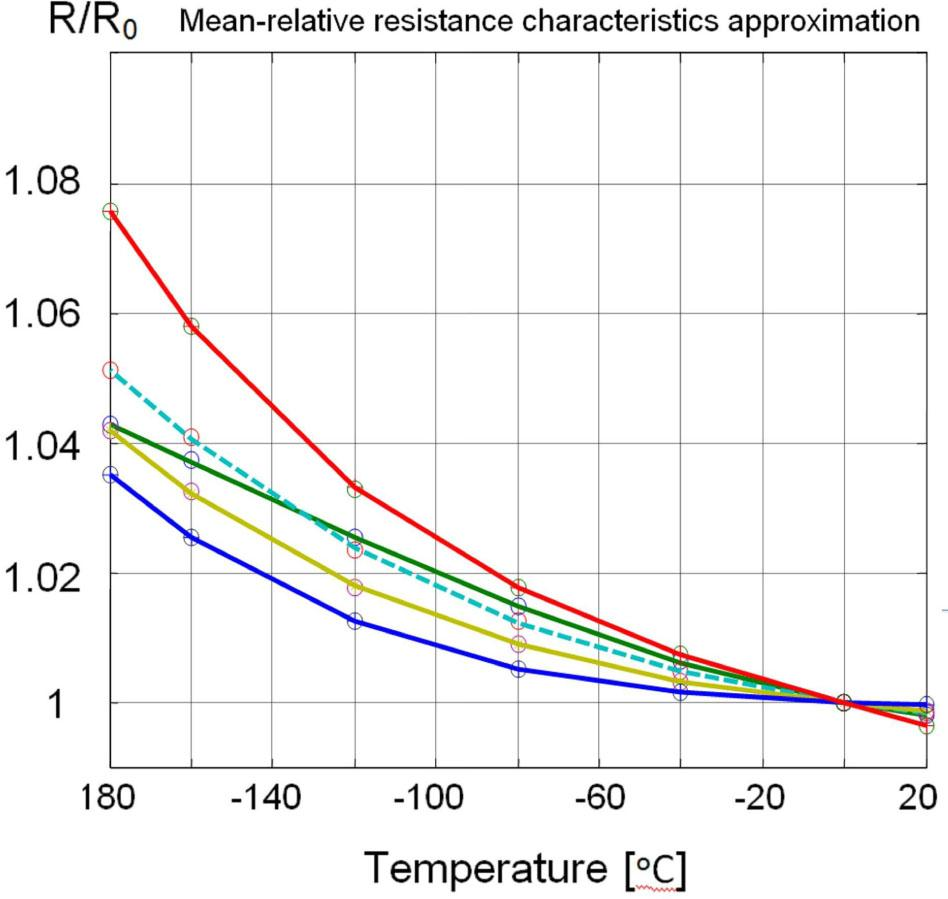Thermal characteristics of relative (normalized) resistance Rr, R323 – green line, R324 – light blue dotted line, R325 – yellow line, DP1931 – blue line, DP1939 – red line.
The modeling method

For the purposes of mathematical modeling the temperature and dimensional dependencies of resistance in analytical forms are needed.

One-dimensional normalized resistance polynomial approximation

As we can see in Fig. 5 and Fig. 6, the characteristics differ between themselves for resistors made of different inks. Therefore they cannot be represented by one universal approximating function. This means that every group of resistors made from a particular resistive ink should be modeled separately. The n–th order polynomial approximating the normalized resistance Rr(T) as a function of temperature can be formulated as:

$Pr(T)=anTn+a(n−1)Tn−1+a(n−2)Tn−2+⋯+a1T+a0$$$\matrix{ {Pr\left( T \right) = {a_n}{T^n} + {a_{\left( {n - 1} \right)}}{T^{n - 1}} + {a_{\left( {n - 2} \right)}}{T^{n - 2}}} \cr { + \cdots + {a_1}T + {a_0}} \cr }$$

where T – temperature (here in °C), aj – j-th polynomial coefficient, n – approximation order.

Because the vector of polynomial coefficients pmean = [a1, a2, a3, . . ., an]T describes completely the normalized resistance Rr(T), our interest is to determine it. The Polyfit Matlab function appeared to be a good choice for this purpose. The approximation order n =4 was chosen to achieve the accuracy better than 0.05 %. For example, the following vector of the mean polynomial coefficients has been obtained: pmean =[a4, a3, a2, a1, a0]T = [17.0623542878488e–012, 554.67022777463 9e–012, 518.760789099527e–009, –20.820560 7094316e–006, 1.00000831544915e+000]T for DP1931 resistors.

The green line in Fig. 7 corresponds to the mean-value of relative resistance approximated by polynomial. As we can see, the accuracy of the approximation is very good. The plots represent averaged characteristics for all 36 resistors on a substrate and for all ten coupons. As can be seen, the dispersion of the normalized resistance value is the largest for the lowest temperature and at –180 °C it is less than 0.4 % of the nominal value 1.035.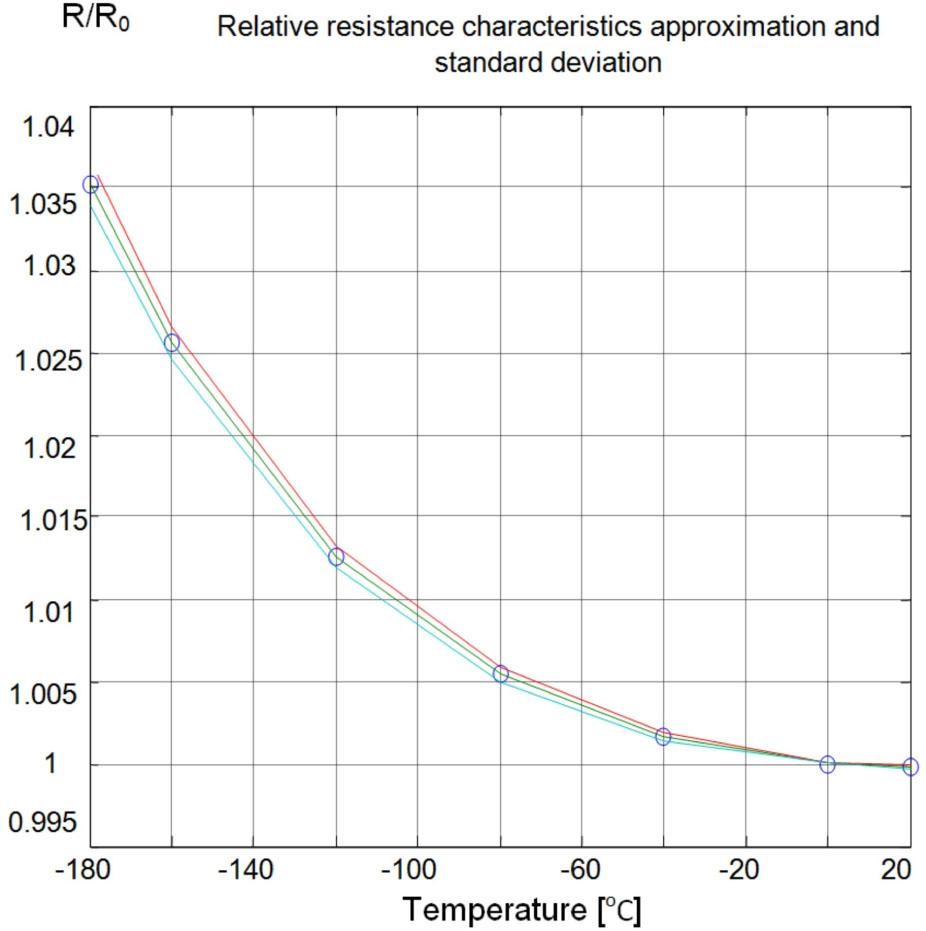Relative resistance Rr vs. temperature and its standard deviation (DP1931), blue circles – mean values of measured relative resistance, red and blue lines – Rr +/-standard deviation of relative resistance, green continuous line – relative resistance characteristics approximated by polynomial.

The resistances can be calculated from the normalized (relative) resistances using the following expression:

$R(T,l,w)=Rr(T,l,w)R0(l,w)$$$R\left( {T,l,w} \right) = Rr\left( {T,l,w} \right){R_0}\left( {l,w} \right)$$

where: Rr(T, l, w) – normalized (relative) resistance, R0(l, w) – resistance at 0 °C.

Assuming that all resistors are governed by the same temperature relation and temperature dependence of their dimensions can be neglected, one obtains:

$R(T,l,w)≈Rr(T)R0(l,w)≈Pr(T)P0(l,w)$$$R\left( {T,l,w} \right) \approx Rr\left( T \right){R_0}\left( {l,w} \right) \approx Pr\left( T \right){P_0}\left( {l,w} \right)$$

where: Pr(T) – 1D-polynomial approximation of normalized resistance temperature dependence, P0(l, w) – 2D-polynomial approximation of R0(l,w).

As we can see, the modeling process consists of two stages. First stage – one-dimensional modeling of temperature relationship Pr(T) ∼ Rr(T) and second stage – 2D approximation process: P0(l,w) ∼ R0(l, w). As the first stage has already been done, the second stage should be solved.

Two-dimensional polynomial approximation

In the expression 5 it is necessary to determine the symbolic two-dimensional function P0(l, w). There are several dimensional modeling methods for description of this two-dimensional relationship. Among them, the Golonka method  improved by Edward and O’Brien  or Stecher  as well as Chebyshev approximation [23, 24] ensure good accuracy. The Golonka and Chebyshev methods were evaluated as the most accurate. However, the optimization process used for Golonka improved functional model identification may converge very slowly because of its multimodality, and sometimes even cannot converge, at all. On the other hand, the Chebyshev method, which provides a unique solution, is very sensitive to moving of its lattice nodes, what in terms of physics means that it is sensitive to the inaccuracy in determining dimensions of the resistors. Therefore, in this work a new two-dimensional polynomial approximation method has been elaborated. This method is similar to the Chebyshev method and ensures better accuracy.

The resistance function R0(l, w) is approximated by the appropriate two-dimensional polynomial:

$P0(l,w)=∑i=0q∑j=0pci,jliwj$$${P_0}\left( {l,w} \right) = \sum\limits_{i = 0}^q {\sum\limits_{j = 0}^p {{c_{i,j}}{l^i}{w^j}} }$$

where: l and w are the length and width of resistor, respectively; p and q are the orders of approximation along the l-axis and w-axis, respectively; ci,j–coefficients, which are to be determined.

Taking the orders of the approximation p and q equal to 5, a vector of coefficients Coeff = [C1, C2, C3, . . ., C36]T of 2D-approximation polynomial is obtained with good accuracy, by solving the system of equations:

$R=P0(l,w)Coef f$$$R = {P_0}\left( {l,w} \right)Coef\,f$$

where: R = [R1, R2, R3, . . ., R36]T – vector of all resistors values counted by rows in the test matrix (Table 1):

$P0(l,w)=[PR1(l,w)PR2(l,w)PR3(l,w)⋮PR36(l,w)]$$${P_0}\left( {l,w} \right) = \left[ {\matrix{ {{P_{R1}}\left( {l,w} \right)} \hfill \cr {{P_{R2}}\left( {l,w} \right)} \hfill \cr {{P_{R3}}\left( {l,w} \right)} \hfill \cr \vdots \hfill \cr {{P_{R36}}\left( {l,w} \right)} \hfill \cr } } \right]$$

the base matrix of dimensional variables, where the vector for each resistor has a symbolic form:

$PRK(l,w)=[1,wk,wk2,wk3,wk4,wk5,lk,lkwk,lkwk2,lkwk3,lkwk4,lkwk5,lk2,lk2wk,lk2wk2,lk2wk3,lk2wk4,lk2wk5,⋯,lk5,lk5wk,lk5wk2,lk5wk3,lk5wk4,lk5wk5]$\eqalign{ & {P_{RK}}\left( {l,w} \right) = [1,{w_k},w_k^2,w_k^3,w_k^4,w_k^5,{l_k},{l_k}{w_k},{l_k}w_k^2, \cr & {l_k}w_k^3,{l_k}w_k^4,{l_k}w_k^5,l_k^2,l_k^2{w_k},l_k^2w_k^2,l_k^2w_k^3,l_k^2w_k^4, \cr & l_k^2w_k^5, \cdots,l_k^5,l_k^5{w_k},l_k^5w_k^2,l_k^5w_k^3,l_k^5w_k^4,l_k^5w_k^5] \cr}

One of exact methods (e.g. Gauss-Jordan or LU-factorization) for solving this system of equations is recommended to obtain the vector of coefficients with high accuracy. Calculations should be performed using double precision data format. Having coefficients determined, all the resistors can be described as functions of their dimensions in a symbolic form.

Estimation of modeling errors

As it was mentioned above, many factors, e.g. film inhomogeneity, interactions within resistor body as well as between resistor body and terminations or substrate, affect R(T) characteristics. Such factors cause small distribution of individual R(T) characteristics for the components with identical planar dimension. This could affect the accuracy of modeling. Relative errors for each resistor at each temperature point was calculated as an average value for 10 elements of population:

$AccR=100(Rcalc−Rmeas)/Rmeas$$$Ac{c_R} = 100\left( {{R_{calc}} - {R_{meas}}} \right)/{R_{meas}}$$

where: AccR – accuracy of R-th resistor in %, Rmeas – measured resistance, Rcalc – resistance calculated from the model.

The results of accuracy calculations were collected in a matrix form AccR(l,w) at each temperature point. Next, for these matrices, the average (S), maximal (Smax) and minimal (Smin) relative errors were calculated for each board from the following formulas:

$S=136∑i=16∑j=16Acc(i,j),Smax=maxi,j Acc(i,j),Smin=mini,j Acc(i,j)$\eqalign{ & S = {1 \over {36}}\sum\limits_{i = 1}^6 {\sum\limits_{j = 1}^6 {Acc\left( {i,j} \right)} } , \cr & {S_{\max }} = \mathop {\max }\limits_{i,j} {\mkern 1mu} \,Acc\left( {i,j} \right), \cr & {S_{\min }} = \mathop {\min }\limits_{i,j} {\mkern 1mu} \,Acc\left( {i,j} \right) \cr}
Results
Resistors made of R323 ink

1D-approximation polynomial: The following vector of the mean polynomial coefficients has been obtained for resistors made of R323 ink (sheet resistance Rsq = 103 Ω/sq): pmean = [a4, a3, a2, a1, a0]T = [–8.40281536226495e–012, –588. 405773049851e–012, 809.406506286434e–00 9, –121.228710347784e–006, 1.000196715883 16e+000]T. It allowed us to create the following one-dimensional polynomial Pr(T) = a4T4+ a3T3+ a2T2+ . . .+ a1T + a0 = –8.40281536 226495e–12T4 – 5.88405773049851e–10T3 + 8.09406506286434e–007T2 – 0.1212287103477 84e–006T + 1.00019671588316e+000.

Applying Horner’s rule for calculating the polynomial, we obtain a computationally efficient form (computer printout): Pr(T)=((((–0.8402815362264953966797291e–11*TEMP–0.5884057730498509150610015e–9)*TEMP+0.8094065062864338502802378e–6)*TEMP–0.1212287103477836179262275e–3)*TEMP+1.000196715883162834614950), where TEMP denotes temperature.

In this way, the number of multiplications was reduced (from 10 to 4) without loss of the accuracy.

2D-approximation polynomial: Next, applying expressions 6 to 9, 2D-approximation polynomial P0(l,w) was calculated (computer printout): P0(l,w)= (83554.20*l+46372.76*w–149786.0*l*w+96290.49*l*wˆ2–28464.94*l*wˆ3+3951.733*l*wˆ4–209.0804*l*wˆ5+135528.3*lˆ2*w–87466.41*lˆ2*wˆ2+25857.34*lˆ2*wˆ3–3585.410*lˆ2*wˆ4+189.4075*lˆ2*wˆ5–50118.21*lˆ3*w+32221.39*lˆ3*wˆ2–9484.827*lˆ3*wˆ3+1309.930*lˆ3*wˆ4–68.96543*lˆ3*wˆ5+7855.159*lˆ4*w–5026.751*lˆ4*wˆ2+1471.770*lˆ4*wˆ3–202.2487*lˆ4*wˆ4+10.60411*lˆ4*wˆ5–443.4330*lˆ5*w+282.2578*lˆ5*wˆ2–82.13061*lˆ5*wˆ3+11.22123*lˆ5*wˆ4–.5855611*lˆ5*wˆ5–29964.13*wˆ2+8880.923*wˆ3–1235.012*wˆ4+65.42886*wˆ5–74090.54*lˆ2+27437.32*lˆ3–4305.576*lˆ4+243.3984*lˆ5-25465.53).

Combining the 1D polynomial Pr (T) with 2D polynomial P0(l, w) the full description of mathematical model was obtained (computer printout): P(T,l,w) = (83554.20*l+46372.76*w–149786.0*l*w+96290.49*l*wˆ2–28464.94*l*wˆ3+3951.733*l*wˆ4–209.0804*l*wˆ5+135528.3*lˆ2*w–87466.41*lˆ2*wˆ2+25857.34*lˆ2*wˆ3–3585.410*lˆ2*wˆ4+189.4075*lˆ2*wˆ5–50118.21*lˆ3*w+32221.39*lˆ3*wˆ2–9484.827*lˆ3*wˆ3+1309.930*lˆ3*wˆ4–68.96543*lˆ3*wˆ5+7855.159*lˆ4*w-5026.751*lˆ4*wˆ2+1471.770*lˆ4*wˆ3–202.2487*lˆ4*wˆ4+10.60411*lˆ4*wˆ5-443.4330*lˆ5*w+282.2578*lˆ5*wˆ2-82.13061*lˆ5*wˆ3+11.22123*lˆ5*wˆ4–.5855611*lˆ5*wˆ5–29964.13*wˆ2+8880.923*wˆ3–1235.012*wˆ4+65.42886*wˆ5–74090.54*lˆ2+27437.32*lˆ3–4305.576*lˆ4+243.3984*lˆ5–25465.53)*((((–0.8402815362264953966797291e–11*TEMP–0.5884057730498509150610015e–9)*TEMP+0.8094065062864338502802378e–6)*TEMP–0.1212287103477836179262275e–3)*TEMP+1.000196715883162834614950).

Using this model the following relative error matrix AccR [%] at 0 °C was obtained:

$|0.0346−0.4396−0.3627−0.06350.02140.02140.2474−0.1748−0.2206−0.02710.02380.02150.36800.0463−0.05120.00790.02540.02150.09320.0169−0.00050.02100.02370.0217−0.2463−0.1089−0.02010.01550.02050.0200−0.3820−0.1827−0.04340.00830.01800.0195|$$$\left| {\matrix{ \hfill {0.0346} & \hfill { - 0.4396} & \hfill { - 0.3627} & \hfill { - 0.0635} & \hfill {0.0214} & \hfill {0.0214} \cr \hfill {0.2474} & \hfill { - 0.1748} & \hfill { - 0.2206} & \hfill { - 0.0271} & \hfill {0.0238} & \hfill {0.0215} \cr \hfill {0.3680} & \hfill {0.0463} & \hfill { - 0.0512} & \hfill {0.0079} & \hfill {0.0254} & \hfill {0.0215} \cr \hfill {0.0932} & \hfill {0.0169} & \hfill { - 0.0005} & \hfill {0.0210} & \hfill {0.0237} & \hfill {0.0217} \cr \hfill { - 0.2463} & \hfill { - 0.1089} & \hfill { - 0.0201} & \hfill {0.0155} & \hfill {0.0205} & \hfill {0.0200} \cr \hfill { - 0.3820} & \hfill { - 0.1827} & \hfill { - 0.0434} & \hfill {0.0083} & \hfill {0.0180} & \hfill {0.0195} \cr } } \right|$$

The average error S(0 °C) = –0.0335 % and maximal and minimal values of relative errors: Smin (0 °C) = –0.4396 %, Smax (0 °C) = 0.3680 % for this matrix are valid. Similar matrices and indicators have been calculated at each temperature point. The plots of dimensional distribution of relative errors and temperature dependence of relative errors are shown in Fig. 8 and Fig. 9, respectively. As we see, the average error is close to zero at all temperature points. However, at a temperature in the vicinity of zero the scattering of the errors is the smallest but it is not equal to zero.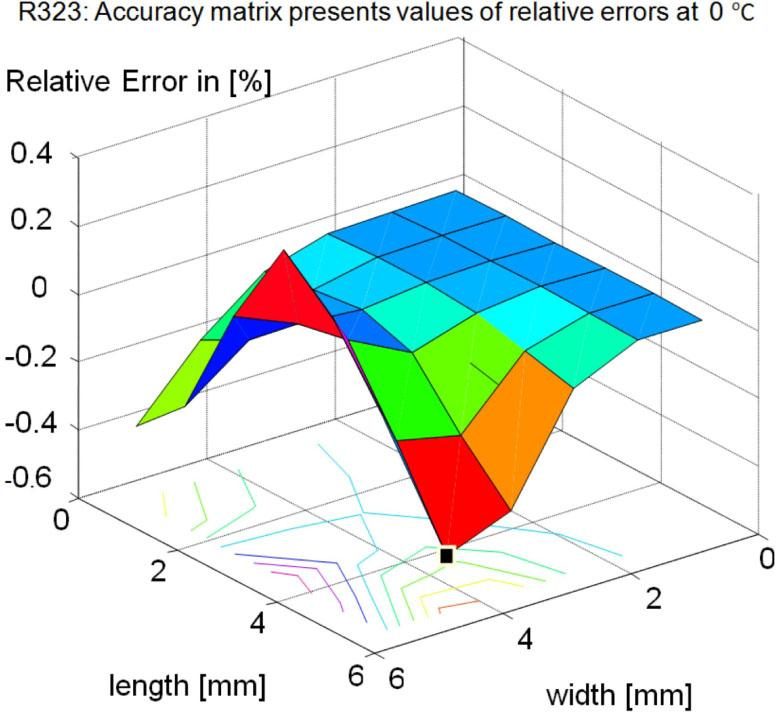Two-dimensional plot of relative errors at 0 °C.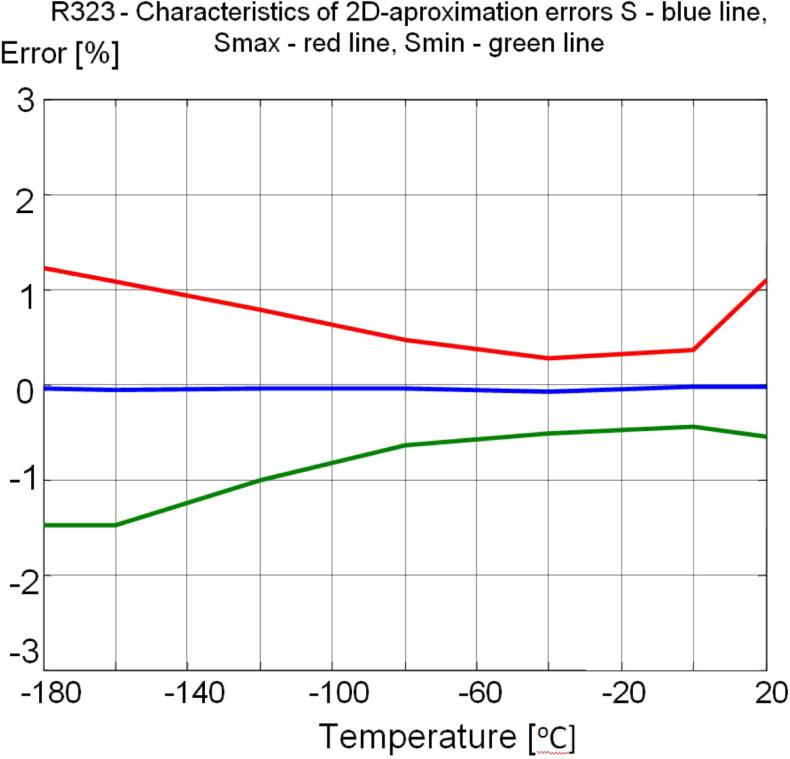Plot of temperature dependence of relative errors, average error – blue line, maximal error – red line, minimal error – green line.

Applying longer integer data format and integer divisions in the polynomial calculations this inaccuracy can be overcome: P(T,l,w) = (5741801237 925429/68719476736*l+3186711816261359/68719476736*w–6999912627694779/274877906944–5146606658744423/34359738368*l*w+827128999799781/8589934592*l*wˆ2–7824383707824711/274877906944*l*wˆ3+1086244226653739/274877906944*l*wˆ4–7356363110292235/35184372088832*l*wˆ5+1164178944500429=8589934592_lˆ2*w–3005322888524689/34359738368*lˆ2*wˆ2+7107612344336475/274877906944*lˆ2*wˆ3–1971099944798019/549755813888*lˆ2*wˆ4+6664185035254301/35184372088832*lˆ2*wˆ5–6888194362096929/137438953472*lˆ3*w+8856948477841461/274877906944*lˆ3*wˆ2–5214338995780667/549755813888*lˆ3*wˆ3+2880565444784041/2199023255552*lˆ3*wˆ4–4853010967155661/70368744177664*lˆ3*wˆ5+4318419510177029/549755813888*lˆ4*w–5526971397073691/1099511627776*lˆ4*wˆ2+6472914700571789/4398046511104*lˆ4*wˆ3–7115991790562483/35184372088832*lˆ4*wˆ4+1492395587381659/140737488355328*lˆ4*wˆ5–7800955237179143/17592186044416*lˆ5*w+1241382780133703/4398046511104*lˆ5*wˆ2–5779427892674011/70368744177664*lˆ5*wˆ3+3158494179461033/281474976710656*lˆ5*wˆ4–5274265191258867/9007199254740992*lˆ5*wˆ5–8236477328720081/274877906944*wˆ2+4882339192415683/549755813888*wˆ3–678954968503345/549755813888*wˆ4+143879592938063/2199023255552*wˆ5–5091463408481717/68719476736*lˆ2+7541912871107027/274877906944*lˆ3–2367015313627021/549755813888*lˆ4+8563819245258181/35184372088832*lˆ5)*((((–0.8402815362264953966797291e–11*TEMP–0.5884057730498509150610015e–9)*TEMP+0.8094065062864338502802378e-6)*TEMP–0.1212287103477836179262275e–3)*TEMP+1.000196715883162834614950);

Using this model the following relative error matrix AccR [%] at 0 °C was obtained:

$|0.01970.01970.01970.01970.01970.01970.01970.01970.01970.01970.01970.01970.01970.01970.01970.01970.01970.01970.01970.01970.01970.01970.01970.01970.01970.01970.01970.01970.01970.01970.01970.01970.01970.01970.01970.0197|$$$\left| {\matrix{ \hfill {0.0197} & \hfill {0.0197} & \hfill {0.0197} & \hfill {0.0197} & \hfill {0.0197} & \hfill {0.0197} \cr \hfill {0.0197} & \hfill {0.0197} & \hfill {0.0197} & \hfill {0.0197} & \hfill {0.0197} & \hfill {0.0197} \cr \hfill {0.0197} & \hfill {0.0197} & \hfill {0.0197} & \hfill {0.0197} & \hfill {0.0197} & \hfill {0.0197} \cr \hfill {0.0197} & \hfill {0.0197} & \hfill {0.0197} & \hfill {0.0197} & \hfill {0.0197} & \hfill {0.0197} \cr \hfill {0.0197} & \hfill {0.0197} & \hfill {0.0197} & \hfill {0.0197} & \hfill {0.0197} & \hfill {0.0197} \cr \hfill {0.0197} & \hfill {0.0197} & \hfill {0.0197} & \hfill {0.0197} & \hfill {0.0197} & \hfill {0.0197} \cr } } \right|$$

The average error: S(0 °C) = 0.0197 %, and maximal and minimal values of relative errors: Smin(0 °C) = 0.0197 %, Smax(0 °C) = 0.0197 % for this matrix are valid. Similar matrices and indicators have been calculated at each temperature point. In this way, we obtained better accuracy in the neighborhood of 0 °C (in the range of temperature from 0 to –60 °C) (Fig. 10 and Fig. 11). Thus, by changing the data format we obtained significant improvement of modeling errors.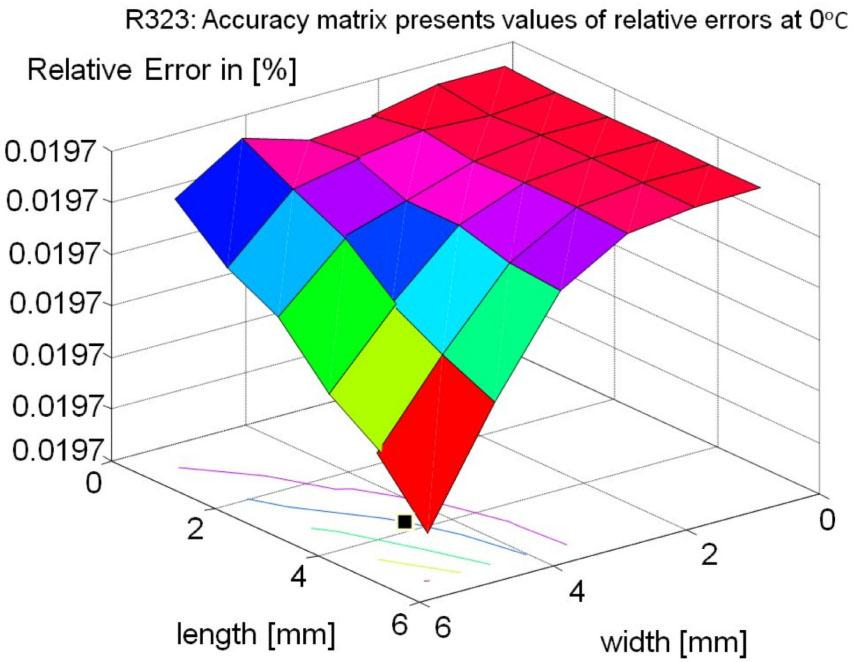Two-dimensional plot of relative errors at 0 °C.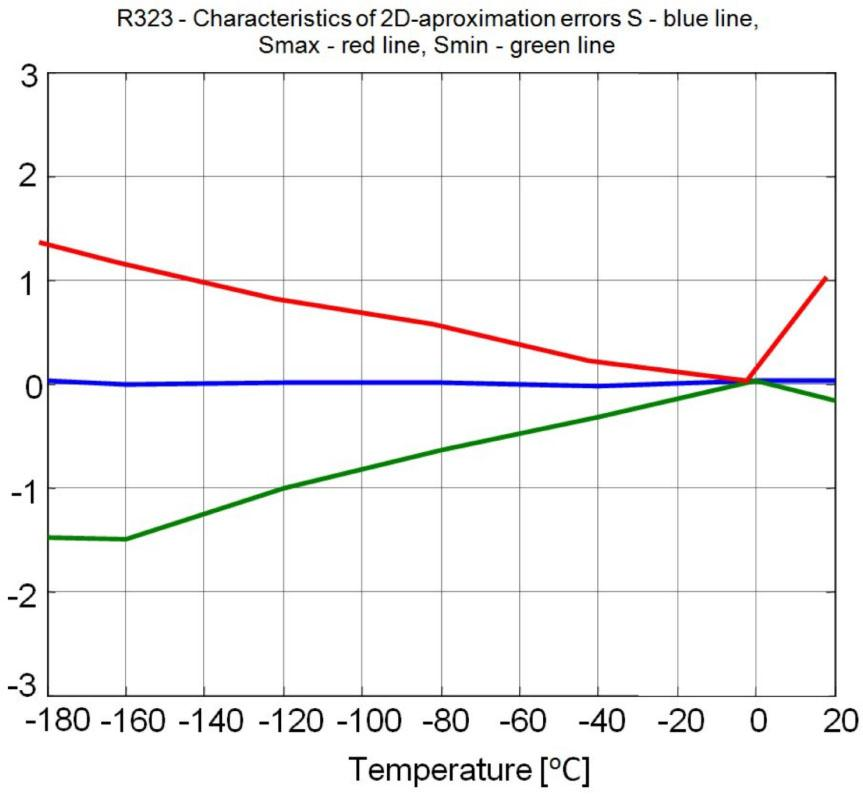Plot of temperature dependence of relative errors, average error – blue line, maximal error – red line, minimal error – green line.
Resistors made of R324 ink

For resistors made of R324 ink (sheet resistance Rsq = 104 Ω/sq) the mathematical model obtained in a similar way as in the case of R323 resistors, showed the following values of accuracy at –120 °C: the average error: S(–120 °C) = –0.0222 %, and maximal and minimal values of relative errors: Smin(–120 °C) = –0.6434 %, Smax(–120 °C) = 0.6974 %. Similar matrices and indicators have been calculated at each temperature point. The relative error matrix AccR [%] at –120 °C is:

$|−0.1825−0.3480−0.1289−0.5565−0.6434−0.2357−0.1824−0.1199−0.0146−0.2953−0.4095−0.32920.0461−0.1668−0.1404−0.2061−0.2281−0.3197−0.1429−0.0435−0.0640−0.1149−0.2180−0.56510.36600.17530.17870.19070.1040−0.21730.68260.66070.69740.66180.59420.2461|$$$\left| {\matrix{ \hfill { - 0.1825} & \hfill { - 0.3480} & \hfill { - 0.1289} & \hfill { - 0.5565} & \hfill { - 0.6434} & \hfill { - 0.2357} \cr \hfill { - 0.1824} & \hfill { - 0.1199} & \hfill { - 0.0146} & \hfill { - 0.2953} & \hfill { - 0.4095} & \hfill { - 0.3292} \cr \hfill {0.0461} & \hfill { - 0.1668} & \hfill { - 0.1404} & \hfill { - 0.2061} & \hfill { - 0.2281} & \hfill { - 0.3197} \cr \hfill { - 0.1429} & \hfill { - 0.0435} & \hfill { - 0.0640} & \hfill { - 0.1149} & \hfill { - 0.2180} & \hfill { - 0.5651} \cr \hfill {0.3660} & \hfill {0.1753} & \hfill {0.1787} & \hfill {0.1907} & \hfill {0.1040} & \hfill { - 0.2173} \cr \hfill {0.6826} & \hfill {0.6607} & \hfill {0.6974} & \hfill {0.6618} & \hfill {0.5942} & \hfill {0.2461} \cr } } \right|$$

The plots of dimensional and temperature dependence of relative errors are shown in Fig. 12 and Fig. 13, respectively. The accuracy of the behavioral model appears to be a little bit worse than for resistors made of R323 ink below –140 °C.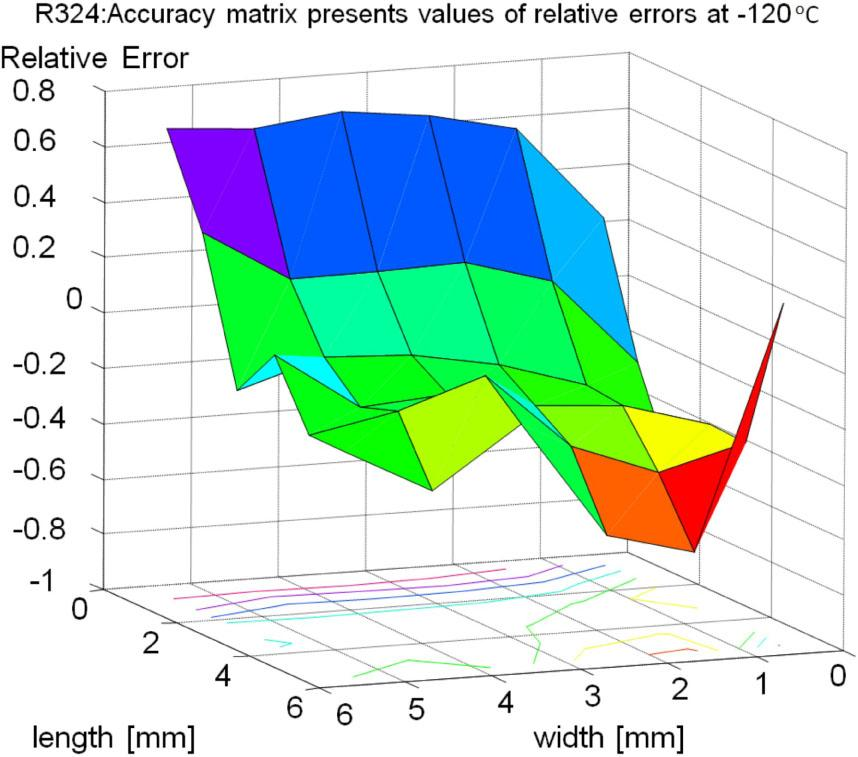Two-dimensional plot of relative errors at –120 °C.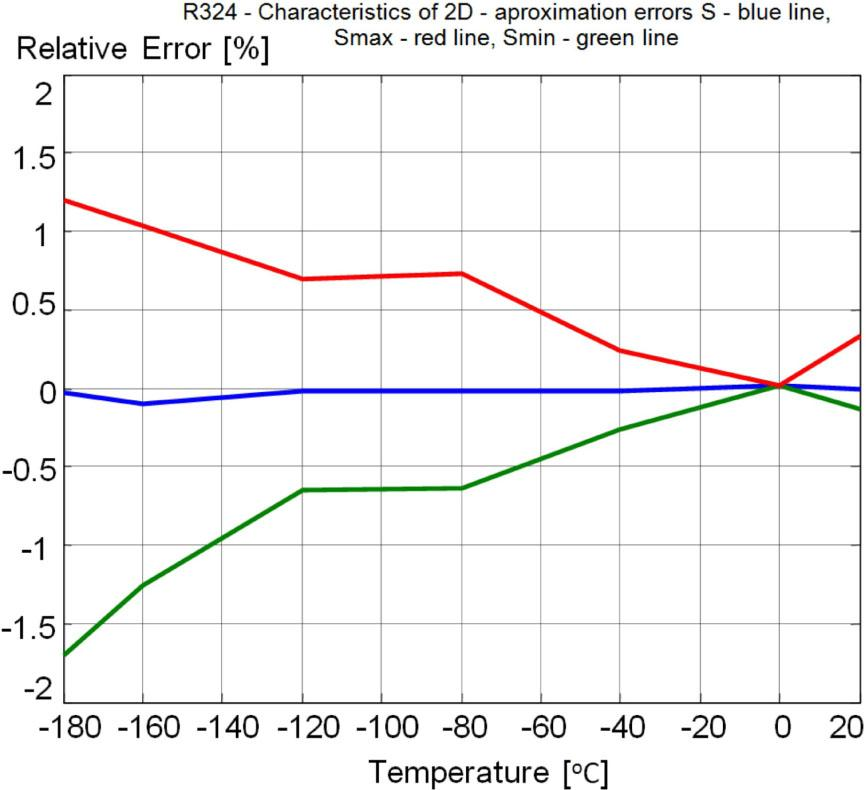Plot of temperature dependence of relative errors, average error – blue line, maximal error – red line, minimal error – green line.
Resistors made of R325 ink

For resistors made of R325 ink (sheet resistance Rsq = 105 W/sq), the relative error matrix AccR [%] at –180 °C is as follows:

$|−0.3838−0.8205−0.3796−0.4278−0.1431−0.0444−0.5326−0.3491−0.2763−0.04660.0400−0.00410.0160−0.3219−0.2008−0.1817−0.1609−0.0231−0.3548−0.1695−0.3825−0.2067−0.28080.06440.35090.12040.17460.10060.14140.23980.74530.92021.02960.96720.78070.3676|$$$\left| {\matrix{ \hfill { - 0.3838} & \hfill { - 0.8205} & \hfill { - 0.3796} & \hfill { - 0.4278} & \hfill { - 0.1431} & \hfill { - 0.0444} \cr \hfill { - 0.5326} & \hfill { - 0.3491} & \hfill { - 0.2763} & \hfill { - 0.0466} & \hfill {0.0400} & \hfill { - 0.0041} \cr \hfill {0.0160} & \hfill { - 0.3219} & \hfill { - 0.2008} & \hfill { - 0.1817} & \hfill { - 0.1609} & \hfill { - 0.0231} \cr \hfill { - 0.3548} & \hfill { - 0.1695} & \hfill { - 0.3825} & \hfill { - 0.2067} & \hfill { - 0.2808} & \hfill {0.0644} \cr \hfill {0.3509} & \hfill {0.1204} & \hfill {0.1746} & \hfill {0.1006} & \hfill {0.1414} & \hfill {0.2398} \cr \hfill {0.7453} & \hfill {0.9202} & \hfill {1.0296} & \hfill {0.9672} & \hfill {0.7807} & \hfill {0.3676} \cr } } \right|$$

The average error: S(–180 °C) = 0.0102 %, minimal and maximal values of relative errors: Smin(–180 °C) = –0.8205 %, Smax(–180 °C) = 1.0296 % for this matrix are valid. Similar matrices and indicators have been calculated at each temperature point.

The plots of dimensional and temperature dependence of relative errors are shown in Fig. 14 and Fig. 15, respectively. The accuracy of the R325 behavioral model appeared to be a little bit better than for resistors made of R323 and R324 inks.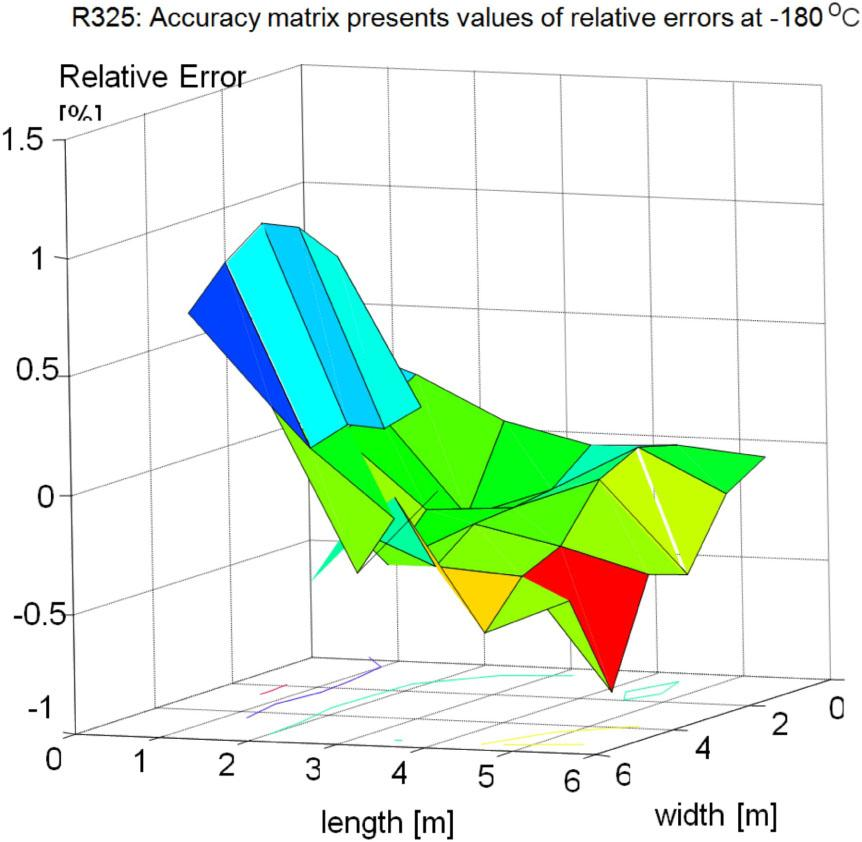Two-dimensional plot of relative errors at –180 °C.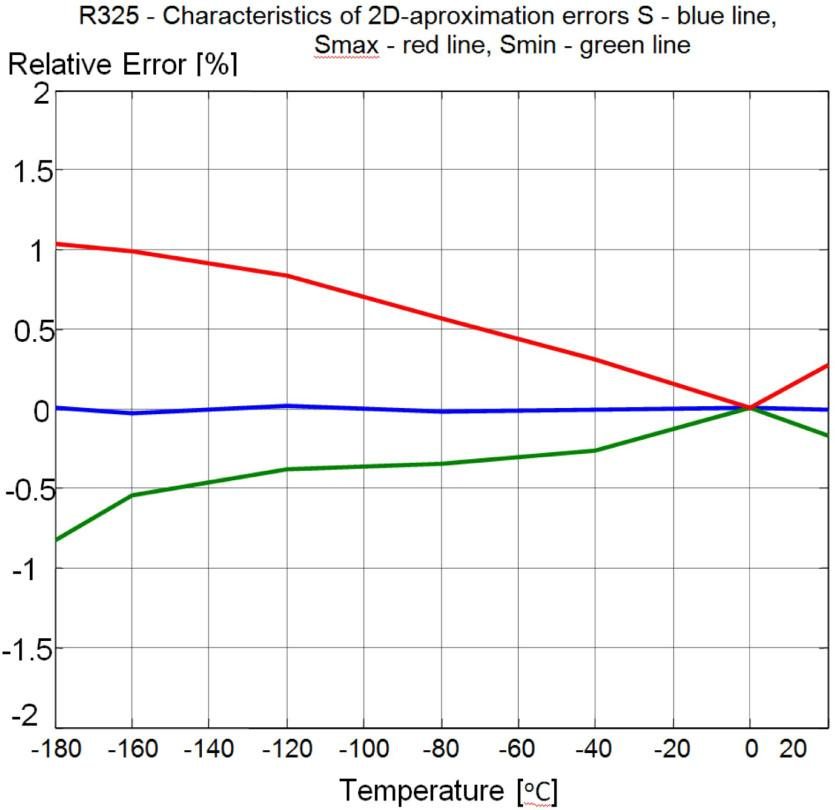Plot of temperature dependence of relative errors, average error – blue line, maximal error – red line, minimal error – green line.
Resistors made of DP1931ink

For resistors made of DP1931 ink (sheet resistance Rsq = 103 Ω/sq) the following mathematical model was obtained: P(T,l,w)=(5539890246818979/34359738368*l+407189429514097/4294967296*w-6980326931738757/137438953472-5126019057908203/17179869184*l*w+6737851480673561/34359738368*l*wˆ2-1074381341501717/17179869184*wˆ2+5183283981166013/274877906944*wˆ3-182745265658445/68719476736*wˆ4+2505450511301973/17592186044416*wˆ5-5033373366850799/34359738368*lˆ2+7768829743601591/137438953472*lˆ3-2592295626746799/274877906944*lˆ4+39010126886843/68719476736*lˆ5-8108637093897161/137438953472*l*wˆ3+4566919509270455/549755813888*l*wˆ4-7816940107805669/17592186044416*l*wˆ5+4726638633140861/17179869184*lˆ2*w-6227558645991857/34359738368*lˆ2*wˆ2+3747519747462157/68719476736*lˆ2*wˆ3-2109662789128353/274877906944*lˆ2*wˆ4+7218129238341703/17592186044416*lˆ2*wˆ5-7282554847041023/68719476736*lˆ3*w+4784959792798973/68719476736*lˆ3*wˆ2-5745978039138385/274877906944*lˆ3*wˆ3+1614557144356367/549755813888*lˆ3*wˆ4-5517215126197517/35184372088832*lˆ3*wˆ5+303539466295757/17179869184*lˆ4*w-3184430773706689/274877906944*lˆ4*wˆ2+3816637668748727/1099511627776*lˆ4*wˆ3-8566554726816813/17592186044416*lˆ4*wˆ4+7310612332166731/281474976710656*lˆ4*wˆ5-584641277978719/549755813888*lˆ5*w+6123643598655569/8796093022208*lˆ5*wˆ2-7327678125959783/35184372088832*lˆ5*wˆ3+8213434998364339/281474976710656*lˆ5*wˆ4-3501782994106985/2251799813685248*1ˆ5*wˆ5)*((((0.1815758610018955456632047e-10TEMP+0.8597882878894229400129041e-9)*TEMP+0.5252185801276130554341446e-6)*TEMP-0.2123441648718566170279953e-4)*TEMP+1.000004314275278582968554).

The relative error matrix AccR [%] at –180 °C is as follows:

$|−0.1261−0.2114−0.1311−0.2050−0.2798−0.2154−0.1627−0.1476−0.1357−0.2164−0.3278−0.20130.0249−0.0997−0.0560−0.0830−0.1235−0.3306−0.04710.05600.03900.0154−0.1616−0.25320.32250.25730.28250.29010.34900.03640.26060.46610.49450.42160.3258−0.1662|$$$\left| {\matrix{ \hfill { - 0.1261} & \hfill { - 0.2114} & \hfill { - 0.1311} & \hfill { - 0.2050} & \hfill { - 0.2798} & \hfill { - 0.2154} \cr \hfill { - 0.1627} & \hfill { - 0.1476} & \hfill { - 0.1357} & \hfill { - 0.2164} & \hfill { - 0.3278} & \hfill { - 0.2013} \cr \hfill {0.0249} & \hfill { - 0.0997} & \hfill { - 0.0560} & \hfill { - 0.0830} & \hfill { - 0.1235} & \hfill { - 0.3306} \cr \hfill { - 0.0471} & \hfill {0.0560} & \hfill {0.0390} & \hfill {0.0154} & \hfill { - 0.1616} & \hfill { - 0.2532} \cr \hfill {0.3225} & \hfill {0.2573} & \hfill {0.2825} & \hfill {0.2901} & \hfill {0.3490} & \hfill {0.0364} \cr \hfill {0.2606} & \hfill {0.4661} & \hfill {0.4945} & \hfill {0.4216} & \hfill {0.3258} & \hfill { - 0.1662} \cr } } \right|$$

The average error: S(–180 °C) = –0.0011 %, minimal and maximal values of relative errors: Smin(–180 °C) = –0.3306 %, Smax(–180 °C) = 0.4945 % for this matrix are valid. Similar matrices and indicators have been calculated at each temperature point. The plots of dimensional and temperature dependence of relative errors are shown in Fig. 16 and Fig. 17, respectively.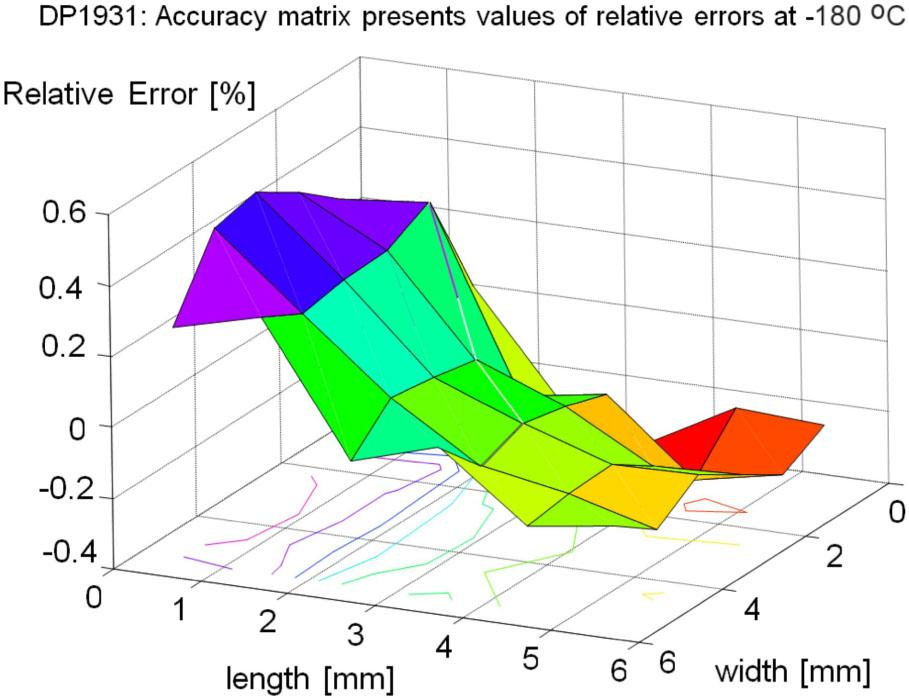Two-dimensional plot of relative errors at –180 °C.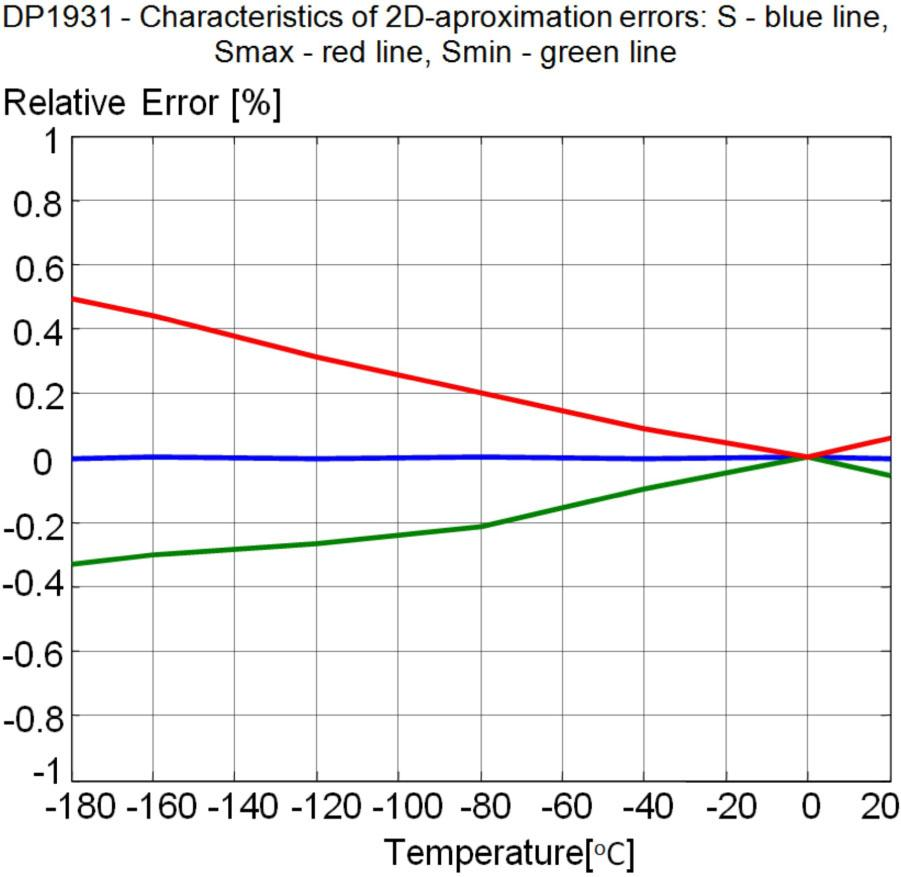Plot of temperature dependence of relative errors, average error – blue line, maximal error – red line, minimal error – green line.
Resistors made of DP1939 ink

For resistors made of DP1939 ink (sheet resistance Rsq = 104 W/sq), the mathematical model, obtained in similar way as in case of resistors made of R323 ink, showed the following values of accuracy at –80 °C: average error: S(–80 °C) = 0.0062 %, minimal and maximal values of relative errors: Smin(–80 °C) = –0.2615 %, Smax(–80 °C) = 0.4313 % for this matrix. The relative error matrix AccR [%] at –180 °C is as follows:

$|−0.1840−0.1961−0.1028−0.2297−0.26150.4161−0.1806−0.1506−0.1884−0.1702−0.07080.4313−0.1392−0.1689−0.0874−0.1761−0.2060−0.0740−0.1065−0.0509−0.0874−0.1479−0.1624−0.21950.07870.02830.03780.0657−0.0271−0.07370.33370.39650.37170.39140.41710.2719|$$$\left| {\matrix{ \hfill { - 0.1840} & \hfill { - 0.1961} & \hfill { - 0.1028} & \hfill { - 0.2297} & \hfill { - 0.2615} & \hfill {0.4161} \cr \hfill { - 0.1806} & \hfill { - 0.1506} & \hfill { - 0.1884} & \hfill { - 0.1702} & \hfill { - 0.0708} & \hfill {0.4313} \cr \hfill { - 0.1392} & \hfill { - 0.1689} & \hfill { - 0.0874} & \hfill { - 0.1761} & \hfill { - 0.2060} & \hfill { - 0.0740} \cr \hfill { - 0.1065} & \hfill { - 0.0509} & \hfill { - 0.0874} & \hfill { - 0.1479} & \hfill { - 0.1624} & \hfill { - 0.2195} \cr \hfill {0.0787} & \hfill {0.0283} & \hfill {0.0378} & \hfill {0.0657} & \hfill { - 0.0271} & \hfill { - 0.0737} \cr \hfill {0.3337} & \hfill {0.3965} & \hfill {0.3717} & \hfill {0.3914} & \hfill {0.4171} & \hfill {0.2719} \cr } } \right|$$

Similar matrices and indicators have been calculated at each temperature point. The plots of dimensional and temperature dependence of relative errors are shown Fig. 18 and Fig. 19. The accuracy of the behavioral model appeared to be a little bit worse than for resistors made of DP1931 ink.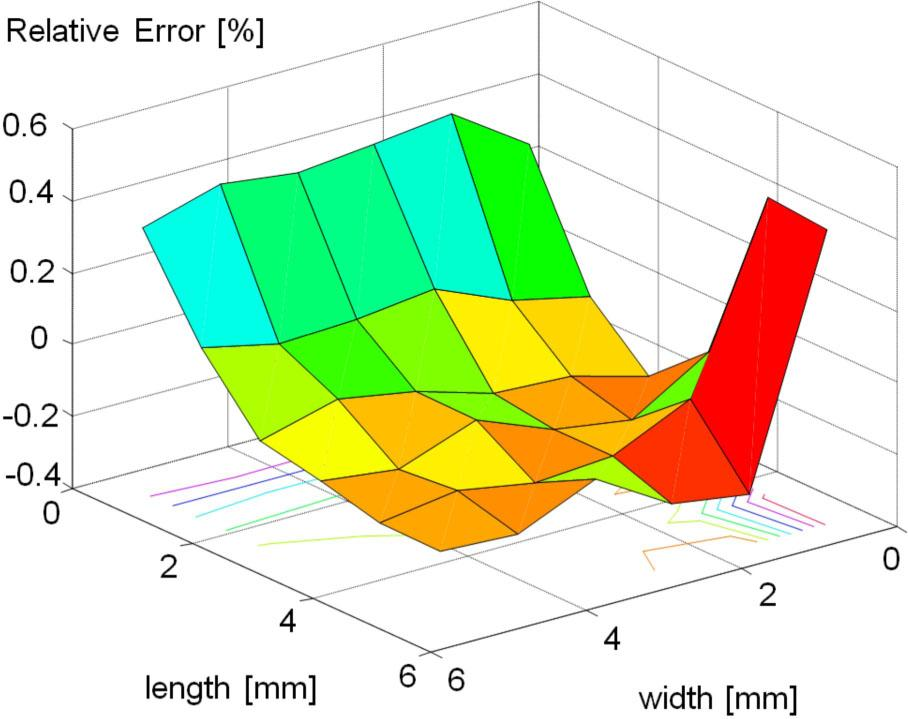Two-dimensional plot of relative errors at –80 °C.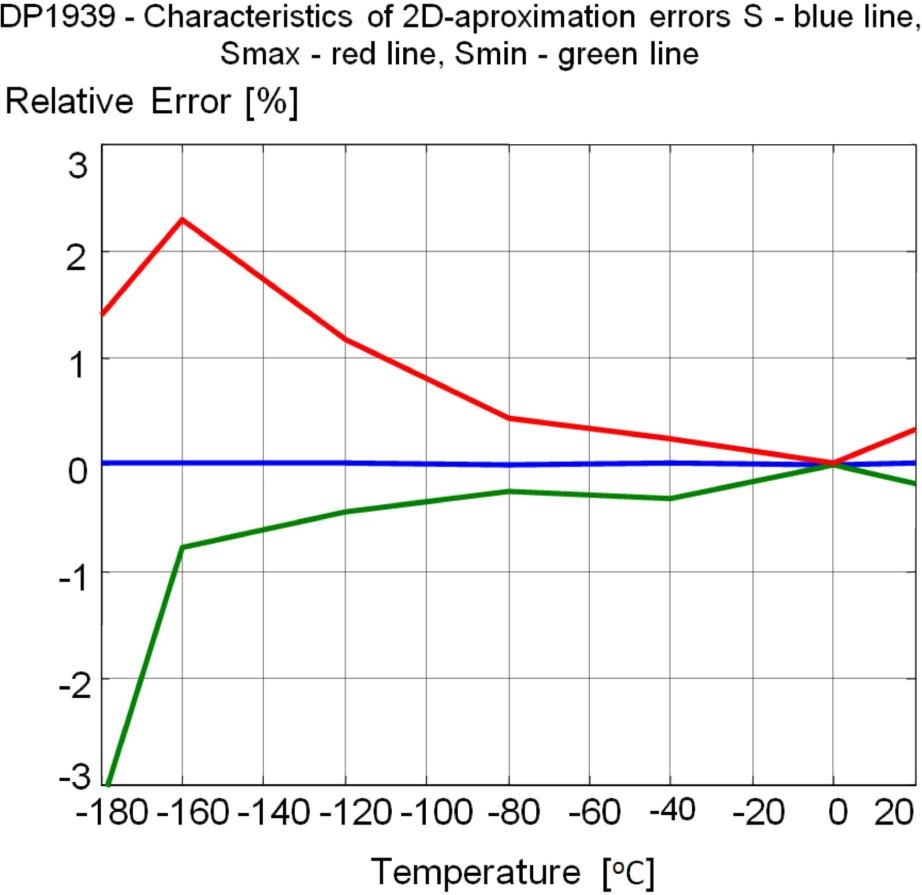Plot of temperature dependence of relative errors, average error – blue line, maximal error – red line, minimal error – green line.

Resuming, one should state that the mean value of modeling errors in all the cases is close to zero. The largest deviations of the modeling errors (Fig. 12, Fig. 14, Fig. 16 and Fig. 18) are appearing in positive direction for short resistors, while for long resistors the errors of modeling are leaning in direction of negative values.

The average dispersion of errors for every group of resistors was estimated at two accuracies of the record of the approximating polynomials: A – the coefficients of P(l,w) polynomial (2D) were recorded as integer divisions and the coefficients of Pr(T) polynomial (1D) had 25 meaningful decimal digits,

B – coefficients of both polynomials P(l,w) and Pr(T) had 7 meaningful decimal digits.

The obtained results were collected in Table 2. Comparing these results one should state that the number of significant digits of the polynomial coefficients has the essential influence on maximum mistakes in resistance determination on the basis of this mathematical model. It can be noticed that the large influence appears particularly in the range of 0 to –80 °C what can be explained by the larger sensitivity of 2D polynomial to the accuracy of the record of its coefficients than that of the 1D polynomial.

Maximal mean errors for examined type of resistors at 0 °C, –80 °C and –180 °C.

S [%] = max{|S|, |Smin|, |Smax|}
DP1931DP1939R323R324R325
ABABABABAB
0 °C8.3· 10−47.860.0180.2800.0191.080.0166.360.0071.54
–80 °C0.217.920.4310.6200.6481.140.7356.550.5671.69
–180 °C0.497.973.1903.2001.4701.441.7026.141.0291.93

Therefore, in this paper we decided to record polynomials as exactly as possible (case A), but our detailed examinations show that taking 15 significant decimal digits for both the polynomials still provides acceptable accuracy.

Conclusions

In this work a new behavioral modeling method of thick-film resistors with a large range of planar dimensions and in a wide temperature range has been presented. This method is based on resistance measurement data. Because the temperature characteristics for resistors made of different inks differ between themselves, they cannot be modeled by one universal mathematical model. Therefore, every type of resistors should be modeled separately.

Based on the experiments and calculations carried out it is possible to state that the presented modeling method has the following features:

its average error of resistance determination is close to zero in the whole studied temperature range for resistors made from different inks,

dispersion of errors for thick-film resistors from various inks and with different dimensions is close to zero in temperatures near zero,

dispersion of errors for thick-film resistors of different types increases while temperature is decreased and below –160 °C it reaches maximal values 1.4 %, 1.7 %, 1 %, 0.5 % and 3.2 % for R323, R324, R325, DP1931, and DP1939, respectively,

the number of meaningful digits of the polynomial coefficients has the essential influence on the maximum error in resistance determination on the basis of this mathematical model. It can be noticed that the influence is the most significant, particularly in the range of temperature of 0 to 80 °C what can be explained by the larger sensitivity to the accuracy of the record of the coefficients of 2D polynomial than that of 1D polynomial,

resistors of higher resistance demonstrate larger increase of negative TCR coefficient than resistors of smaller resistance values,

modeling using this method has to be performed only ones for a given type of resistors,

application of long data format and integer numerical operations can significantly improve the accuracy of calculations.

Outlining the above remarks it should be stated, that the proposed method of dimensional and temperature modeling of thick-film resistors ensures good accuracy for all examined types of resistors and can be used for analysis and design purposes. The introduced mathematical model was exploited while working out the behavioral circuit model for SPICE – the electronic circuit design system .

Considering the problem of modeling of small electronic components working at cryogenic temperatures one should also have in mind the following factors:

measuring methods should not introduce large errors (e.g. one should carry out repeated measurements at one point with the averaging);

calculations should be made with the accuracy as large as possible (selection of so-called exact computational methods, the format of numerical data possible long – double precision, applying the Horner’s rule). The worked out models concern thick-film resistors with a wide range of dimensions. we suppose, that for chosen groups of resistors, e.g. the power resistors, these models will be simpler and not less accurate.

#### Matrix of resistances for every test coupon at each temperature.

[mm]w = 5.91w = 5.19w = 3.96w = 2.51w = 1.31w = 0.59
l = 5.91R1(T)R2(T)R3(T)R4(T)R5(T)R6(T)
l = 5.19R7(T)R8(T)R9(T)R10(T)R11(T)R12(T)
l = 3.96R13(T)R14(T)R15(T)R16(T)R17(T)R18(T)
l = 2.51R19(T)R20(T)R21(T)R22(T)R23(T)R24(T)
l = 1.31R25(T)R26(T)R27(T)R28(T)R29(T)R30(T)
l = 0.59R31(T)R32(T)R33(T)R34(T)R35(T)R36(T)

#### Maximal mean errors for examined type of resistors at 0 °C, –80 °C and –180 °C.

S [%] = max{|S|, |Smin|, |Smax|}
DP1931DP1939R323R324R325
ABABABABAB
0 °C8.3· 10−47.860.0180.2800.0191.080.0166.360.0071.54
–80 °C0.217.920.4310.6200.6481.140.7356.550.5671.69
–180 °C0.497.973.1903.2001.4701.441.7026.141.0291.93

Pierce J.W., Kuty D.W., Larry J.R., 3rdEuropean Hybrid Microelectronic Conference, Avignon, 1981, p. 283.PierceJWKutyDWLarryJR3rd European Hybrid Microelectronic ConferenceAvignon1981283Search in Google Scholar

Dziedzic A., Microelectron Reliab, 42 (2002), 709.DziedzicAMicroelectron Reliab42200270910.1016/S0026-2714(02)00044-6Search in Google Scholar

Tugnawat Y., Kuhn W., 12thNASA Symposium on VLSI Design, Coer d’Alene, Idaho, 2005, p. 1.TugnawatYKuhnW12th NASA Symposium on VLSI DesignCoer d’Alene, Idaho20051Search in Google Scholar

Buchanan E.D., Benford D.J., Forgione J.B., Moseley S.H., Wollack E.J., Cryogenics, 52 (2012), 550.BuchananEDBenfordDJForgioneJBMoseleySHWollackEJCryogenics52201255010.1016/j.cryogenics.2012.06.017Search in Google Scholar

Pike G.E., Seager C.H., J. Appl. Phys., 48 (1977), 5152.PikeGESeagerCHJ. Appl. Phys481977515210.1063/1.323595Search in Google Scholar

Halder N.C., Electrocomp. Sci. Technol., 11 (1983), 21.HalderNCElectrocomp. Sci. Technol1119832110.1155/APEC.11.21Search in Google Scholar

Licznerski B.W., 5thEuropean Hybrid Microelectronics Conference, Stresa, 1985, p. 389.LicznerskiBW5th European Hybrid Microelectronics ConferenceStresa1985389Search in Google Scholar

Dziedzic A., Golonka L.J., Licznerski B.W., 7thCzechoslovak Conference on Electronics and Vacuum Physics, Bratislava, 1985, p. 855.DziedzicAGolonkaLJLicznerskiBW7th Czechoslovak Conference on Electronics and Vacuum PhysicsBratislava1985855Search in Google Scholar

Chiou B.-S., Sheu J.-Y., J. Electron. Mater., 21 (1992), 575.ChiouB-SSheuJ-YJ. Electron. Mater21199257510.1007/BF02655423Search in Google Scholar

Kusy A., Physica B, 240 (1997), 226.KusyAPhysica B240199722610.1016/S0921-4526(97)00426-2Search in Google Scholar

Crosbie G.M., Johnson M., Trela W., J. Appl. Phys., 84 (1998), 2913.CrosbieGMJohnsonMTrelaWJ. Appl. Phys841998291310.1063/1.368447Search in Google Scholar

Golonka L.J., Dziedzic A., Henke M., 43rdInternational Colloquium, Vol. 2, Ilmenau, 1998, p. 203.GolonkaLJDziedzicAHenkeM43rd International ColloquiumVol. 2Ilmenau1998203Search in Google Scholar

Yang P., Rodriguez M.A., Kotula P., Miera B.K., Dimos D., J. Appl. Phys., 89 (2001), 4175.YangPRodriguezMAKotulaPMieraBKDimosDJ. Appl. Phys892001417510.1063/1.1353563Search in Google Scholar

Woodcraft A.L., Sudiwala R.V., Wakui E., Paine C., J. Low Temp. Phys., 134 (2004), 925.WoodcraftALSudiwalaRVWakuiEPaineCJ. Low Temp. Phys134200492510.1023/B:JOLT.0000013209.08494.01Search in Google Scholar

Lake Shore Cryotronics, Inc., Lstc Appendixd_1.Pdf, Www.Lakeshore.Com.Lake Shore Cryotronics, IncLstc Appendixd_1.PdfWww.Lakeshore.ComSearch in Google Scholar

Cosmo De V., Gush H., Halpern M., Leung A., Rev. Sci. Instrum., 58 (1987), 441.Cosmo DeVGushHHalpernMLeungARev. Sci. Instrum58198744110.1063/1.1139251Search in Google Scholar

Jacob J.M., Application and Design with Analog Integrated Circuits, A Prentice-Hall Co., Reston, Virginia, 1982.JacobJMApplication and Design with Analog Integrated CircuitsA Prentice-Hall CoReston, Virginia1982Search in Google Scholar

Balik F., Sommer W., Elektronika, 51 (3) (2011), 84.BalikFSommerWElektronika513201184Search in Google Scholar

Goryachev M., Galliou S., Imbaud J., Abbe P.H., Cryogenics, 6 (2013), 1.GoryachevMGalliouSImbaudJAbbePHCryogenics620131Search in Google Scholar

Golonka L.J., Int. J. Hybri. Microelectron., 4 (1981), 405.GolonkaLJInt. J. Hybri. Microelectron41981405Search in Google Scholar

Edward L.N.M., O’Brien T.G., Microelectronics Conference, Melbourne, 1991, p. 112.EdwardLNMO’BrienTGMicroelectronics ConferenceMelbourne1991112Search in Google Scholar

Stecher G., Hybri. Circ., 17 (1988), 24.StecherGHybri. Circ1719882410.1108/eb042473Search in Google Scholar

Balik F., Golonka L.J., Jurewicz R.J., 15th Conference ISHM-Poland, Cracow, 1991, p. 5.BalikFGolonkaLJJurewiczRJ15th Conference ISHM-PolandCracow19915Search in Google Scholar

Balik F., Golonka L.J, Jurewicz R.J., Transactions of the Technical University of Kosice, 3 (1993), 106.BalikFGolonkaLJJurewiczRJTransactions of the Technical University of Kosice31993106Search in Google Scholar

Microsim Pspice A/D, Circuit Analysis Reference Manual, MicroSim Co., 1995.Microsim Pspice A/DCircuit Analysis Reference ManualMicroSim Co1995Search in Google Scholar

• #### Growth, spectral, optical, dielectric and third order nonlinear studies on 2-amino 4-methylpyridinium salicylate single crystals

Recommended articles from Trend MD